Computational Resources

This section provides information about the computational resources required to use PROC TRANSREG.

Let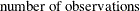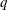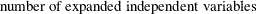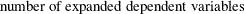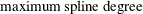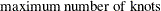More than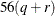plus the maximum of the data matrix size, the optimal scaling work space, and the covariance matrix size bytes of array space are required. The data matrix size is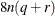bytes. The optimal scaling work space requires less than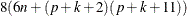bytes. The covariance matrix size is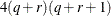bytes.

PROC TRANSREG tries to store the original and transformed data in memory. If there is not enough memory, a utility data set is used, potentially resulting in a large increase in execution time. The amount of memory for the preceding data formulas is an underestimate of the amount of memory needed to handle most problems. These formulas give the absolute minimum amount of memory required. If a utility data set is used, and if memory can be used with perfect efficiency, then roughly the amount of memory stated previously is needed. In reality, most problems require at least two or three times the minimum.

PROC TRANSREG sorts the data once. The sort time is roughly proportional to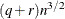.

One regression analysis per iteration is required to compute model parameters (or two canonical correlation analyses per iteration for METHOD=CANALS). The time required to accumulate the crossproducts matrix is roughly proportional to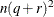. The time required to compute the regression coefficients is roughly proportional to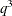.

Each optimal scaling is a multiple regression problem, although some transformations are handled with faster special-case algorithms. The number of regressors for the optimal scaling problems depends on the original values of the variable and the type of transformation. For each monotone spline transformation, an unknown number of multiple regressions is required to find a set of coefficients that satisfies the constraints. The B-spline basis is generated twice for each SPLINE and MSPLINE transformation for each iteration. The time required to generate the B-spline basis is roughly proportional to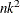.